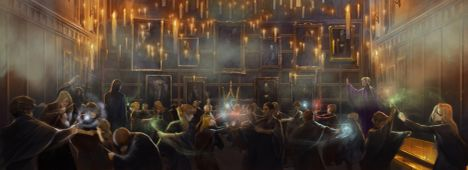# Wizard DuelrebornNational Girls' Programmi...13/16/38
Limits 1s, 512 MBWizard Duel is approaching soon in Hogwarts and the students of the Dueling Club are absolutely thrilled! One more time, they can show off their skills in front of the whole school in a fun, yet sincere competition against each other. It is a competition where in each round, a wizard/witch faces off against another wizard/witch in a friendly battle of witchcraft and wizardry.

Hermione, being the clever girl she is, wants to pick her battles carefully. Each wizard and witch has a fixed power in her eyes. The value of the power of any wizard, she couldn’t determine. But what she does know, is the ratio of power between a few pairs of wizards and/or witches. She is wondering, given the information she has, if it is possible to know that in a battle of one wizard vs another, who will win?

Formally, there are $N$ wizards and witches (including Hermione). Each of them has $P_i$ fixed powers, but that value is unknown to Hermione. She does know, however, that the powers are positive and the ratio of $P_i$ and $P_j$ (i.e. $\frac{p_i}{p_j}$) for $M$ pairs of wizards and witches $(i, j)$.

Hermione has $Q$ queries, each of them asks that in a battle of wizard $i$ and wizard $j$, is it possible to know who will win given the information she has? If yes, then who wins? Is it a draw instead?

Note that, a wizard $i$ will win against another wizard $j$ if the former has more power i.e. $P_i > P_j$. And they will draw if they have equal power i.e. $P_i = P_j$.

## Input

The first line of input contains an integer $T (1 \le T \le 100)$ denoting the number of test cases.

For each test case, the first line contains three integers $N, M, Q$.

• $2 \le N \le 35$.

• $1 \le M, Q \le \frac{N(N-1)}{2}$.

$M$ lines follow, each containing four integers $i, j, x, y$ meaning that the ratio of power between wizards $i$ and $j$ is $\frac{x}{y}$ (i.e. $\frac{P_i}{P_j} = \frac{x}{y}$). You can safely assume that $i \neq j$. Furthermore, the values $x$ and $y$ will be co-prime to each other i.e. $gcd(x, y)=1$. You can also assume that each of the information regarding the ratio of powers will be consistent throughout a test case. No two unordered $(i, j)$ pair will be the same in a test case.

• $1 \le i, j \le N$ and $i \neq j$.

• $1 \le x, y \le 3$ and $gcd(x,y) = 1$.

Another $Q$ lines follow, each containing two integers $a, b$ describing the queries. For each of these queries, Hermione wants to know who will win in the battle between wizards $a$ and $b$.

• $1 \le a, b \le N$ and $a \neq b$.

## Output

For each query, output a string on a line. For a query $(a, b)$, you should output —

• win” if wizard $a$ will win against $b$.

• lose” if wizard $a$ will lose against $b$.

• draw” if the wizards have equal power and will draw.

• unknown” if it cannot be decided who will win or draw, based on the information Hermione has.

Please note that, you should print these strings without the quotes. Refer to the sample I/O for clarity.

## Sample

InputOutput
2
4 2 3
1 2 2 3
3 2 2 1
1 2
3 1
2 4
2 1 1
1 2 1 1
2 1

lose
win
unknown
draw


#### Explanation for the Sample I/O

The first three lines in output correspond to the queries of test case $1$. The last line corresponds to the query of test case $2$.

In test case $1$,

• $\frac{P_1}{P_2} = \frac{2}{3}$, thus wizard $1$will lose against wizard $2$.

• $\frac{P_3}{P_1} = 3$, thus wizard $3$ will win against wizard $1$.

• there is no information about wizard $4$, thus it is unknown who will win between wizards $2$ and $4$.

In test case $2$,

• Wizard $1$ and $2$ have equal power, thus it will be a draw.### Submit